# Jeu de taquin

(diff) ← Older revision | Latest revision (diff) | Newer revision → (diff)
Jump to: navigation, search

The French name of a puzzle game introduced in France by H.E. Lucas. It consists of fifteen wooden squares, numbered from one to fifteen, which can be moved in a-box. A single space is free, and must be used to restore the consecutive numbering of the smaller squares. This game is still on sale with some variations (a typical example is the "puzzle" program distributed with the Macintosh operating system).

By analogy, this same name has been given by M.-P. Schützenberger (cf. [a4], [a5]) to a graphical representation of the plactic, or Knuth, congruences.

The Robinson–Schensted construction (cf. Robinson–Schensted correspondence) establishes a bijection between a permutation and a pair of standard Young tableaux (cf. Young tableau and [a2]). It is a fundamental tool in the combinatorics of representations of symmetric groups (cf. [a3]). This construction has been extended to words (i.e. allowing repetitions) by D. Knuth. In the latter case, the first tableau is no longer standard, but semi-standard (the entries are increasing along the columns and non-decreasing along the rows). Knuth has also shown that two words correspond to the same semi-standard tableau if and only if it is possible to obtain one from the other by a succession of certain commutations of letters.

Further, A. Lascoux and Schützenberger have established that the equivalence relation generated by this set of relations is compatible with concatenation. The quotient monoid (which is in bijection with the set of semi-standard tableaux) is known as the plactic monoid. They have also given the fundamental properties of this monoid, in [a1].

The plactic (or Knuth) congruences are the following: Let the alphabetbe totally ordered and suppose that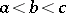. Then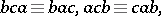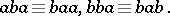This equivalence can be transferred to (semi-standard) skew Young tableaux (cf. Skew Young tableau) in the following way. Two skew Young tableaux are equivalent if and only if one can be obtained from the other by a succession of local transformations corresponding, respectively, to the four plactic congruences: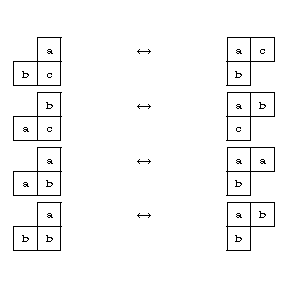Figure: j110030a

Under this equivalence relation each class of skew Young tableaux contains exactly one Young tableau. This statement is in fact equivalent to Knuth's theorem. This game of transformation of tableaux is what is called jeu de taquin.

It can be used to provide an alternative to the original Schensted insertion algorithm as generalized by Knuth. Letbe a word. Consider any skew Young tableau such that, reading its entries from left to right and from bottom to top, one obtains precisely. Then apply jeu de taquin transformations to that tableau a Young tableau is obtained. This last tableau depends only on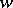, but not on the choices of starting tableau and of transformations. It is exactly the first tableau of the pair associated to.

For example, start with. One can produce the following sequence of transformations. Note that the starting tableau has the "reading property" mentioned above.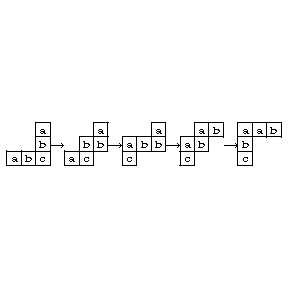Figure: j110030b